Importance: Medium ✭✭
 Author(s): Samal, Robert
 Subject: Graph Theory » Coloring » » Homomorphisms
 Keywords: Clebsch graph cut-continuous mapping edge-coloring homomorphism pentagon
 Posted by: Robert Samal on: July 13th, 2007
Conjecture   Ifis a cubic graph not containing a triangle, then it is possible to color the edges ofby five colors, so that the complement of every color class is a bipartite graph.

This conjecture has several reformulations: the conclusion of the conjecture can be replaced by either of the following:

\itemhas a homomorphism to the Clebsch graph. \item there is a cut-continuous mapping fromto.

For the latter variant, few definitions are in place. A cut-continuous mapping from a graph~to a graph~is a mappingsuch that the preimage of every cut in~is a cut in~. Here, by a cut in~we mean the edge-set of a spanning bipartite subgraph of~---less succinctly, it is the set of all edges leaving some subset of vertices of~.

Cut-continuous mappings are closely related with graph homomorphisms (see [DNR], [S]). In particular, every homomorphism from~to~naturally induces a cut-continuous mapping from~to~; thus, the presented conjecture can be thought of as a weaker version of Nesetril's Pentagon problem.

We mention a generalization of the conjecture, that deals with longer cycles/larger number of colors. The-dimensional projective cube, denoted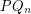, is the simple graph obtained from the-dimensional cube~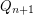by identifying pairs of antipodal vertices (vertices that differ in all coordinates). Note thatis the Clebsch graph.

Question   What is the largest integerwith the property that all cubic graphs of sufficiently high girth have a homomorphism to?

Again, the question has several reformulations due to the following simple proposition.

Proposition   For every graphand nonnegative integer, the following properties are equivalent.
\item There exists a coloring of~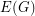by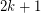colors so that the complement of every color class is a bipartite graph. \itemhas a homomorphism to\itemhas a cut-continuous mapping to~There are high-girth cubic graphs with the largest cut of size less then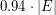. Such graphs do not admit a homomorphism tofor any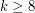, so there is indeed some largest integer~in the above question. To bound this largest~from below, recall that every cubic graph maps homomorphically to. Moreover, it is known [DS] that cubic graphs of girth at least 17 admit a homomorphism to(the Clebsch graph). This shows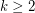(and also provides a support for the main conjecture).

## Bibliography

[DNR] Matt DeVos, Jaroslav Nesetril and Andre Raspaud: On edge-maps whose inverse preserves flows and tensions, \MRref{MR2279171}

*[DS] Matt Devos, Robert Samal: \arXiv[High Girth Cubic Graphs Map to the Clebsch Graph}{math.CO/0602580}

[S] Robert Samal, On XY mappings, PhD thesis, Charles University 2006, tech. report

* indicates original appearance(s) of problem.